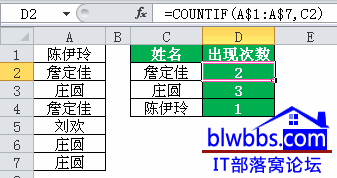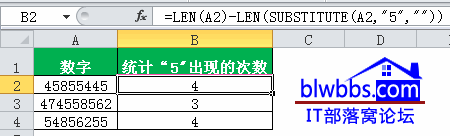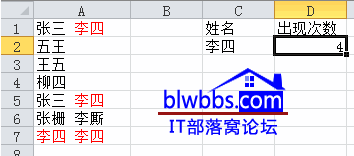# excel计算出现次数的思路和公式

[日期：2012-02-18]   来源：IT部落窝  作者：IT部落窝   阅读：2646[字体： ]

excel计算出现次数实例一：

excel计算出现次数，根据具体的内容和要求不同，方法也不同。

下面是一个基础的excel计算出现次数用法，统计C2：C4单元格在A列出现次数。

D2单元格输入公式：=COUNTIF(A\$1:A\$7,C2)，下拉就OK。这是一个计算出现次数的基础用法。excel计算出现次数实例二：

A列的数字，需要统计数字“5”在单元格出现的次数，比如5在A2单元格出现了几次，在A3单元格又出现了几次。

B2单元格输入公式：=LEN(A2)-LEN(SUBSTITUTE(A2,"5",""))，下拉就可以计算出现次数。

公式思路：用LEN函数计算A1单元格的字节长度，然后利用Substitute函数，把要统计的字符数”5”替换为空，即删除，再次统计这个单元格的字节长度，两次统计结果的差正好是删除的字符数，结果正好等于要查找的字符在单元格内的出现次数。excel计算出现次数实例三：

计算下面A列“李四”出现的次数，注意姓名出现次数（同一个单元格可能多次）。

D2单元格公式为：=SUMPRODUCT((LEN(A1:A7)-LEN(SUBSTITUTE(A1:A7,C2,"")))/LEN(C2))
或者是下面任意数组公式均可：
=SUM(MMULT(LEN(A1:A7)-LEN(SUBSTITUTE(A1:A7,C2,"")),1))/TEXT(LEN(C2),"[=0]1;0")
=COUNT(0/(MID(A1:A7,COLUMN(1:1),LEN(D2))=TEXT(D2,"0;@")))分享到： QQ空间 新浪微博 腾讯微博 人人网